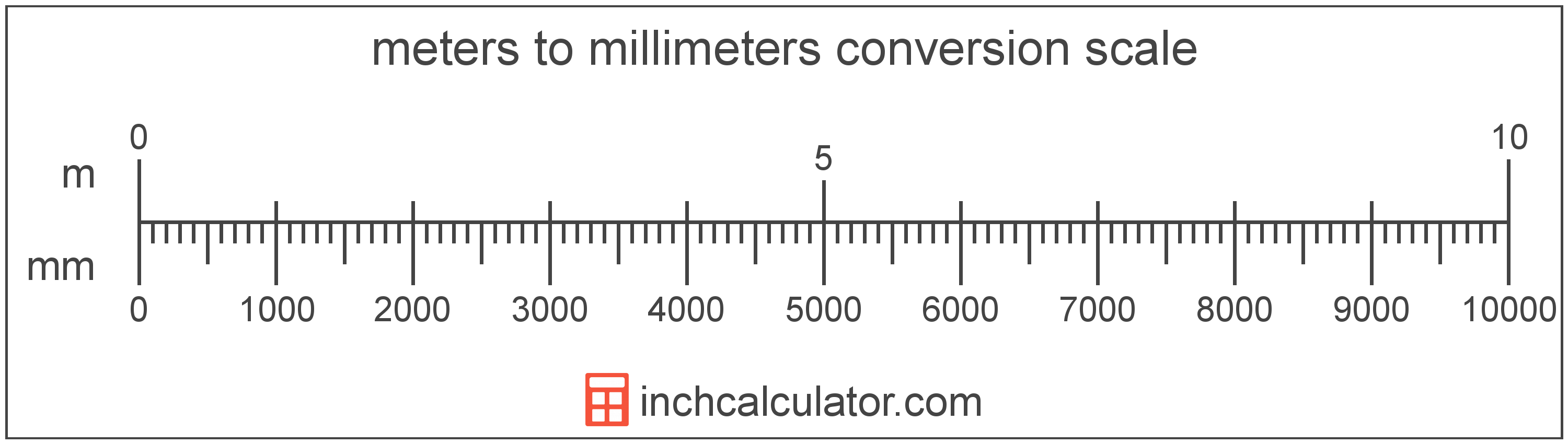# Meters to Millimeters Conversion

Enter the length in meters below to get the value converted to millimeters.

Results in Millimeters:1 m = 1,000 mm
1 m = 100 cm

## How to Convert Meters to MillimetersTo convert a meter measurement to a millimeter measurement, multiply the length by the conversion ratio.

Since one meter is equal to 1,000 millimeters, you can use this simple formula to convert:

millimeters = meters × 1,000

The length in millimeters is equal to the meters multiplied by 1,000.

For example, here's how to convert 5 meters to millimeters using the formula above.
5 m = (5 × 1,000) = 5,000 mm

### How Many Millimeters are in a Meter?

There are 1,000 millimeters in a meter, which is why we use this value in the formula above.

1 m = 1,000 mm

Our inch fraction calculator can add meters and millimeters together, and it also automatically converts the results to US customary, imperial, and SI metric values.

## Meters

The meter is defined as the length of the path traveled by light in vacuum during a time interval with a duration of 1/299,792,458 of a second, according to the most recent 2019 definition. One meter is equal to 100 centimeters or 39.37 inches.

The meter, or metre, is the SI base unit for length in the metric system. Meters can be abbreviated as m; for example, 1 meter can be written as 1 m.

## Millimeters

One millimeter is equal to one-thousandth (1/1,000) of a meter, which is defined as the distance light travels in a vacuum in a 1/299,792,458 second time interval.

The millimeter, or millimetre, is a multiple of the meter, which is the SI base unit for length. In the metric system, "milli" is the prefix for 10-3. Millimeters can be abbreviated as mm; for example, 1 millimeter can be written as 1 mm.

Millimeters are often represented by the smallest ticks on most metric rulers. To get a reference point of the size, the thickness of a US dime is 1.35mm.

We recommend using a ruler or tape measure for measuring length, which can be found at a local retailer or home center. Rulers are available in imperial, metric, or combination with both values, so make sure you get the correct type for your needs.

Need a ruler? Try our free downloadable and printable rulers, which include both imperial and metric measurements.

## Meter to Millimeter Conversion Table

Meter measurements converted to millimeters
Meters Millimeters
0.001 m 1 mm
0.002 m 2 mm
0.003 m 3 mm
0.004 m 4 mm
0.005 m 5 mm
0.006 m 6 mm
0.007 m 7 mm
0.008 m 8 mm
0.009 m 9 mm
0.01 m 10 mm
0.02 m 20 mm
0.03 m 30 mm
0.04 m 40 mm
0.05 m 50 mm
0.06 m 60 mm
0.07 m 70 mm
0.08 m 80 mm
0.09 m 90 mm
0.1 m 100 mm
0.2 m 200 mm
0.3 m 300 mm
0.4 m 400 mm
0.5 m 500 mm
0.6 m 600 mm
0.7 m 700 mm
0.8 m 800 mm
0.9 m 900 mm
1 m 1,000 mm

## References

1. International Bureau of Weights and Measures, The International System of Units, 9th Edition, 2019, https://www.bipm.org/documents/20126/41483022/SI-Brochure-9-EN.pdf
2. United States Mint, Coin Specifications, https://www.usmint.gov/learn/coin-and-medal-programs/coin-specifications